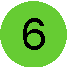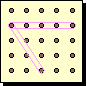# Geoboard

Objective: Place an object on a grid, using columns and rows.
Materials: Geoboards, elastics, colour tiles, screen (to hide board from opponent).Begin by placing an elastic along each row and column of pegs on your geoboard. This will create a grid. In each of the 16 spaces on the grid place one colour tile (red, green, or yellow). Play the game with a partner. Your partner will ask about the colour of tile in a given location on the geoboard (Example "Is the tile in the third column, fourth row green?"). Your job is to answer your partner's yes/no questions until s/he has replicated your design. Switch roles and let your partner hide 16 tiles for you to figure out. If you wish, keep track of the number of guesses you needed and the number your partner needed to correctly reveal each other's boards. The player requiring the fewest number of guesses is the winner. To make the game more difficult, allow players to use blue colour tiles as well, or leave some spaces blank! Make the game easier by arranging tiles in a pattern, or using only 2 colours.

Objective: Relate perimeter and area of rectangles, using manipulatives and diagrams.
Materials: Geoboards, elastics, paper, pencil.

 On a geoboard, to find the perimeter of a rectangle you can count the number of spaces between pins along the path of the elastic. To find the area you multiply the number of spaces between pins on two adjacent sides of the rectangle. The smallest rectangle you can make is actually a square with area of 1 and perimeter of 4. See if you can find each of the following: Find two rectangles with the same perimeter, but different areas. Can you find another pair with the same perimeter, but different areas? Find two rectangles with the same area, but different perimeters. How many figures (squares and rectangles) can you create that have a perimeter equal to their area? How many different sized squares and rectangles can be made on the geoboard (no diagonals!).Objective: Estimate and measure angles, using a circular protractor.
Materials: Geoboards, elastics, circular protractor.You can make a variety of different angles on the geoboard by connecting three points as shown. Try to connect three points to create angles with the following measures: 45o, 90o, 25o, 50o, 110o, 180o, 135o How many different sized angles (less than 90o) can you create on a geoboard? Where would you place the elastics to create the smallest possible angle on the geoboard?